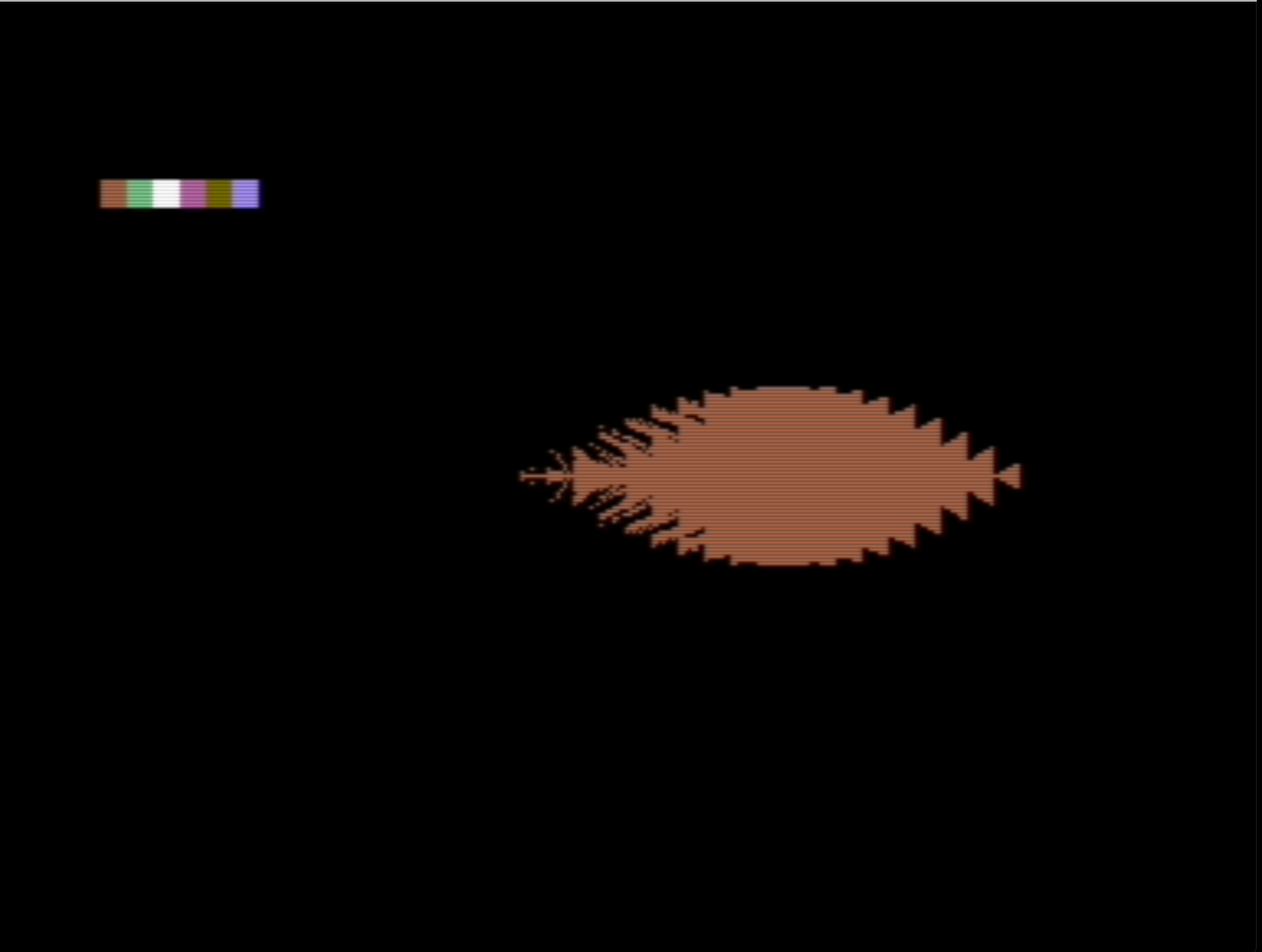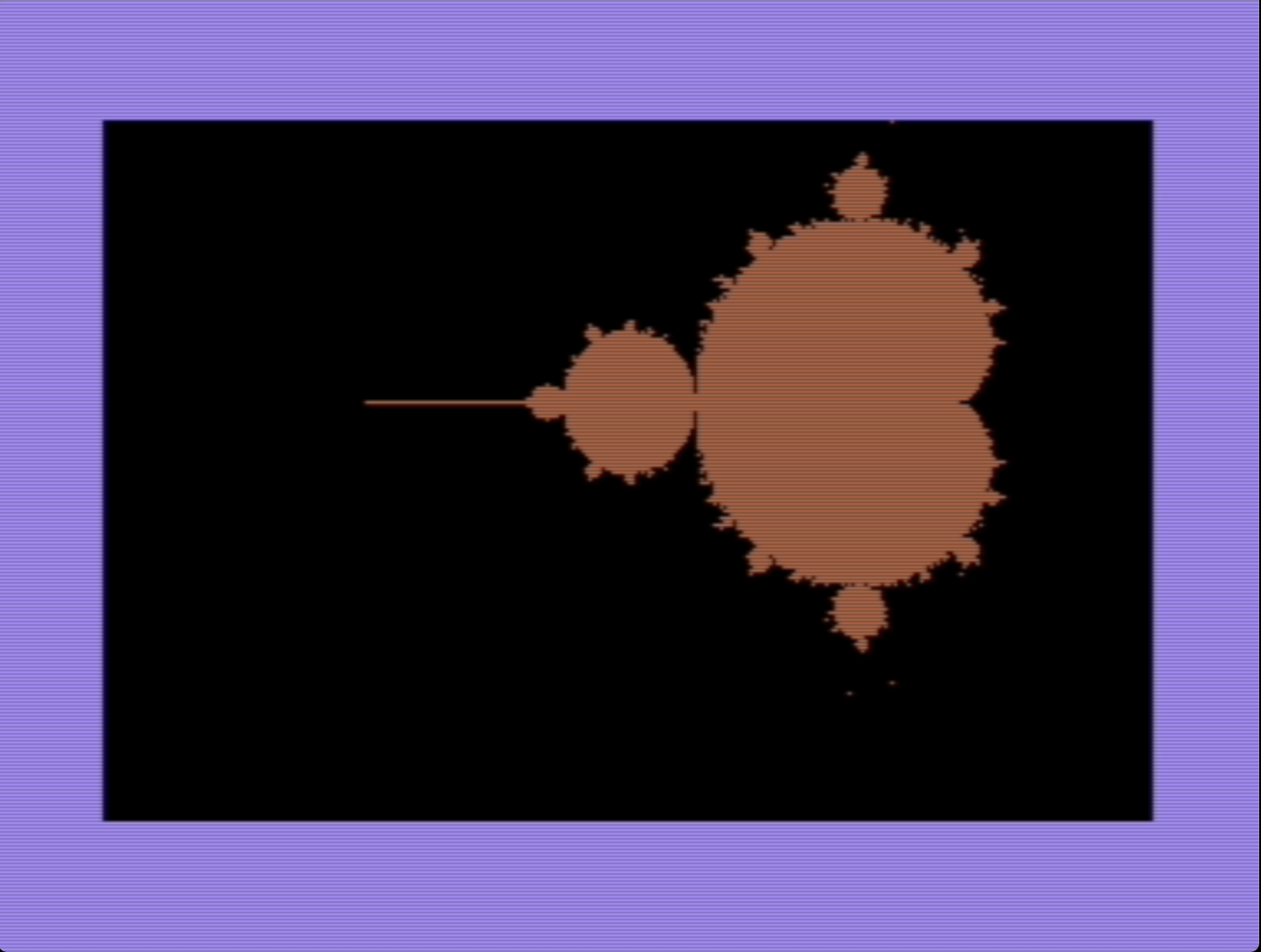I recently recovered the code I wrote on the commodore 64 when I was 10 or so. Among it was a mandelbrot generator, in basic.

``````    5 poke 53265,59:poke 53272,29
6 for i=8192 to 8192+7999:poke i,0:next
10 print"{clr}"
20 for i=200 to 999
30 for j=0 to 7
40 for k=7 to 0 step -1
50 b=2.5-(5/200)*j-8*int(i/40)*(5/200)
60 a=-4+(5/320)*k+(i-int(i/40)*40)*8*(5/320)
70 a1=a:b1=b:co=0
80 a1=a1^2+b1^2+a:b1=2*a1*b1+b
90 d=sqr(a1^2+b1^2)
100 if d>2 then 130
110 if co=5 then gosub 300:goto 130
120 co=co+1:goto 80
130 next k:next j:next i
140 end
300 poke 8192+i*8+j,peek(8192+i*8+j)or 2^k
310 return
600 a=a^2+b^2:b=2*a*b
700 a=a+rc:b=b+ic
800 d=sqr(a^2+b^2)
``````

For some reason or another that I didn’t want to explore, it produced a broken image.It looks like a combination of poor indexing in the graphical memory space and a wrong equation. So I rewrote it, also simplifying the cycle and the “graphical driver”

``````    5 poke 53265,59:poke53272,29
6 fori=8192 to 8192+7999:pokei,0:next
7 print chr\$(147)
10 rs=-3:re=1:is=-1:ie=1.5:rd=(re-rs)/320:id=(ie-is)/200
20 for co=0 to 319
30 for ro=0 to 199
50 r=rs+co*rd:i=is+ro*id
55 it=0:a=0:b=0
57 a1=a^2-b^2+r:b1=2*a*b+i
59 if (a1^2+b1^2)>4 then goto 130
61 a=a1:b=b1:it=it+1
63 if it < 100 then goto 57